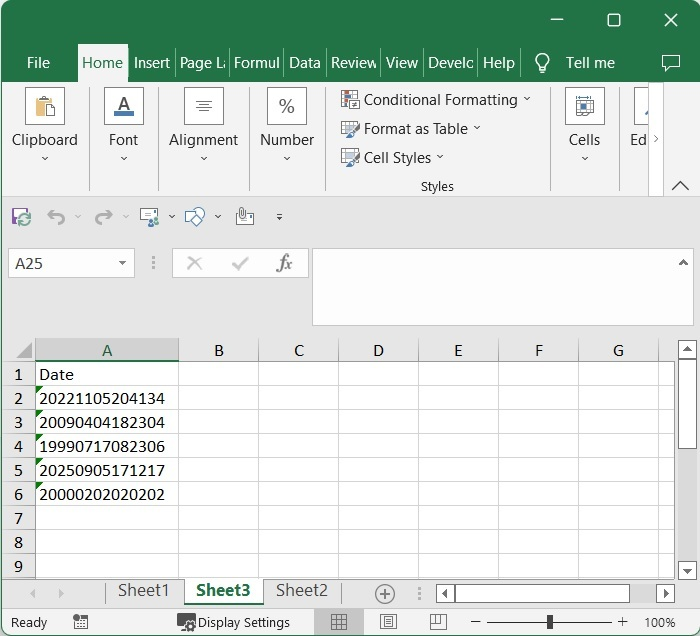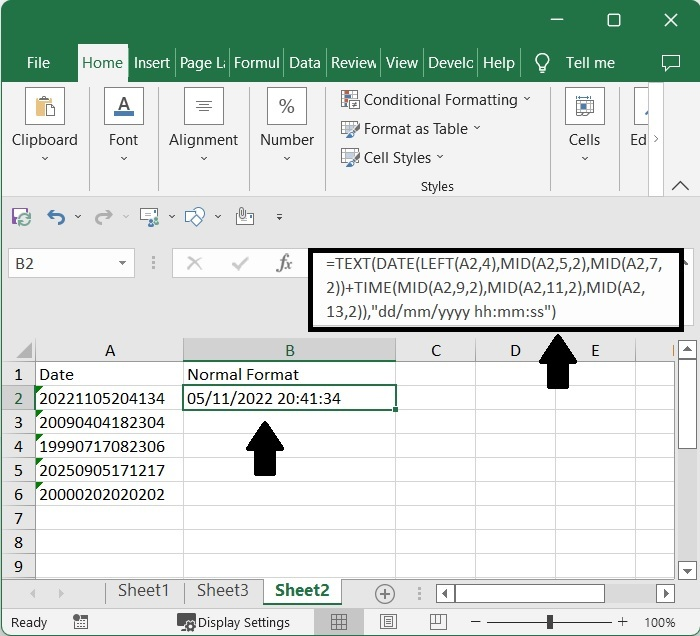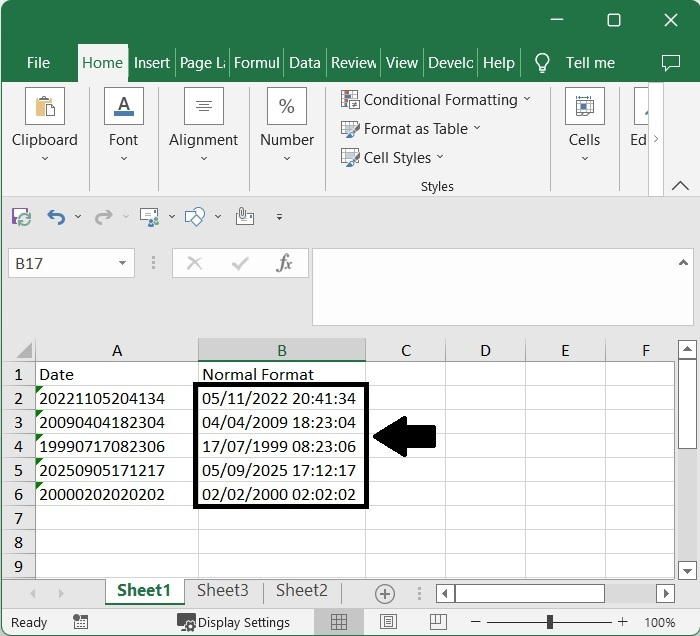# How to Convert yyyymmddhhmmss Date Format to Normal Datetime in Excel?Datetime in Excel?

In Excel, we always try to show the date and time in the format of dd-mm-yyyy hh:mm:ss, which is the standard format when we use the date and time in Excel. But when the date and time are given in another format, some users may feel uncomfortable using them because they could have only used the standard format all this time. Generally, in Excel, when we enter any format of date and time in the sheet, it will be directly converted to the standard format. If we try to convert any other format to standard format manually, then it can be a time-consuming and inaccurate process, as we need to change every date manually.

Read this tutorial to learn how you can convert YYYYMMDDHHMMSS format to a standard format in Excel. We can complete the task using the formulas supported in Excel.

## Converting "yyyymmddhhmmss" Date Format to Normal Datetime

Here, we will first use the formula to get any one result, then use the auto-fill handler to get all the results. Let's take a look at a simple procedure for converting YYYY-MM-DD format to normal date format in Excel.

### Step 1

Consider an excel sheet where the data is a list of dates represented in the format "yyyymmddhhmmss", as shown in the image below.Now, in cell B2, click on an empty cell and enter the formula as follows −

=TEXT(DATE(LEFT(A2,4),MID(A2,5,2),MID(A2,7,2))+TIME(MID(A2,9,2),MID(A2,11,2),MID(A2,13,2)),"dd/mm/yyyy hh:mm:ss")


and Click Enter to get our first result, which looks similar to the below image. In the formula, A2 is the address of the cell where our value is present on the sheet.

Empty cell > Formula > Enter### Step 2

Then, using the auto-fill handle, drag from the first result to all the other results, and our final result will look like the image below.Note − We can also use the formula as =--TEXT(A2,"0000\-00\-00\ 00\:00\:00") but we need to format the cells to complete the task.

## Conclusion

In this tutorial, we used a simple example to demonstrate how to convert yyyymmddhhmmss format to normal format in Excel.